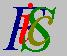Mauro Murzi's pages on Philosophy of Science - Quantum mechanics
Partial derivativesDerivative State description in classical mechanics# [2. Mathematical elements.]

## Partial derivatives.

Let φ (xy) be a complex function of two real variables. As an example, let φ (xy) = e i x y. There are two different rates of change of this function, one with respect to the x variable, the other with respect to the y variable. The first rate of change is determined considering the y variable as a constant and calculating the usual derivative of φ . This is called the first partial derivative of φ (xy) with respect to x and is denoted by ∂φ (xy) / ∂x. In the example we have ∂φ (xy) / ∂x = ∂e i x y / ∂x = y e i (xy + π/2) .

There exists also the first partial derivative of φ (xy) with respect to y, calculated assuming x constant, and denoted by ∂φ (xy) / ∂y. Continuing the example: ∂φ (xy) / ∂ y = ∂e i x y / ∂y = x e i (xy + π/2) .

The second partial derivatives of φ (xy) with respect to x and y is the first partial derivative of ∂φ (xy) / ∂x with respect to y. It is denoted by ∂²φ (xy) / ∂x ∂y and is calculated in the following way:

1. Consider y as a constant and determine the derivative of φ (xy) with respect to x.
2. Consider x as a constant in the derivative and calculate another derivative with respect to y.

A simple example: let z = x3 y. We have:

• z / ∂x = 3 x² y
• ∂²z / ∂x ∂y = 3 x²
• z / ∂y = x 3
• ∂²z / ∂y ∂x = 3 x²

Note that, in the example, ∂²z / ∂x ∂y = ∂²z / ∂y ∂x. In this article are mainly used function satisfying this relation, although it does not hold in general.Derivative State description in classical mechanics